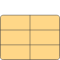# CoilDesign

Calculation of winding parameters (wire diameter, number of turns et al.) and recalculation with optionally chosen parameters; to be adapted to particular design tasks# Information

This information is part of the Modelica Standard Library maintained by the Modelica Association.

This model example shows dimensioning of a winding (wire diameter, number of turns) based on desired operating conditions (voltage, temperature, current density, conductor filling factor) for a given cross-section area of the winding. It can be modified according to the parameters given and sought after for a particular design project.

The calculated winding resistance and number of turns can be used as input parameters to the electrical subsystem of a device to be modelled. Operating voltage V_op can be minimum, nominal and maximum voltage respectively as specified for a particular design project. In conjunction with the setting of the operating temperature T_op, this enables for analysis of the device under worst-case conditions (e.g., minimum required magnetomotive force, maximum allowed ohmic losses, minimum and maximum force respectively).

For manufacturing of a winding, the obtained wire diameter d_wireCalculated must be rounded to that of an available wire. In order to analyse the influence of this rounding, one can enter the chosen wire diameter d_wireChosen and number of turns N_chosen as optional input. Calculation of the resulting winding parameters enables for comparison with the ones obtained otherwise.

# Parameters (23)

rho_20 Value: 0.0178e-6 Type: Resistivity (Ω·m) Description: Resistivity of conductor material at 20 degC (default: Copper) Value: 0.0039 Type: LinearTemperatureCoefficient (¹/K) Description: Temperature coefficient of conductor material's resistivity at 20 degC (default: Copper) Value: 293.15 Type: Temperature (K) Description: Operating temperature of winding Value: rho_20 * (1 + alpha_20 * (T_op - (20 - Modelica.Constants.T_zero))) Type: Resistivity (Ω·m) Description: Resistivity at operating temperature Value: Type: Length (m) Description: Height of winding cross-section Value: Type: Length (m) Description: Width of winding cross-section Value: h_w * b_w Type: Area (m²) Description: Cross-section area of winding Value: Type: Length (m) Description: Average length of one turn Value: Type: Voltage (V) Description: Operating voltage (nominal/ minimum/ maximum voltage depending on design objective) Value: 4e6 Type: CurrentDensity (A/m²) Description: DESIRED current density at operating temperature and voltage resp. Value: 0.6 Type: Real Description: CHOSEN conductor filling factor = total conductor area without insulation/ total winding area Value: V_op / (rho * l_avg * J_desired) Type: Real Description: CALCULATED number of turns Value: sqrt(4 * A_w * c_condFillChosen / (pi * N_calculated)) Type: Diameter (m) Description: CALCULATED wire diameter (without insulation) Value: pi * d_wireCalculated ^ 2 / 4 Type: Area (m²) Description: Calculated wire cross-section area Value: rho * N_calculated * l_avg / A_wireCalculated Type: Resistance (Ω) Description: Winding resistance at operating temperature and voltage resp. with CALCULATED number of turns and wire diameter Value: V_op ^ 2 / R_calculated Type: Power (W) Description: Winding's ohmic losses at operating temperature and voltage resp. with CALCULATED number of turns and wire diameter Value: d_wireCalculated Type: Diameter (m) Description: CHOSEN available wire diameter (without insulation) Value: N_calculated Type: Real Description: CHOSEN number of turns Value: pi * d_wireChosen ^ 2 / 4 Type: Area (m²) Description: Wire cross-section area resulting from CHOSEN wire diameter Value: rho * N_chosen * l_avg / A_wireChosen Type: Resistance (Ω) Description: Winding resistance at operating temperature and voltage resp. resulting from CHOSEN number of turns and wire diameter Value: V_op ^ 2 / R_actual Type: Power (W) Description: Winding's ohmic losses at operating temperature and voltage resp. resulting from CHOSEN number of turns and wire diameter Value: V_op * 4 / (R_actual * pi * d_wireChosen ^ 2) Type: CurrentDensity (A/m²) Description: Current density at operating temperature and voltage resp. resulting from CHOSEN number of turns and wire diameter Value: N_chosen * pi * d_wireChosen ^ 2 / (4 * A_w) Type: Real Description: Conductor filling factor resulting from CHOSEN number of turns and wire diameter